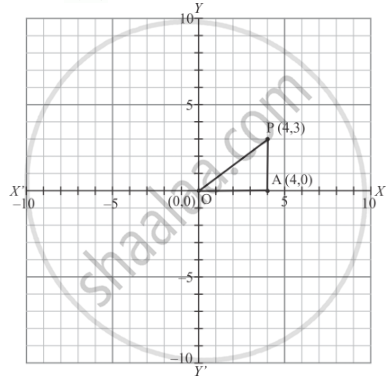# The Distance of the Point P (4, 3) from the Origin is - Mathematics

MCQ

The distance of the point P (4, 3) from the origin is

• 4

• 3

• 5

• 7

#### Solution

The point  P(4,3) is shown in the graph given below:In ΔOAPis right angled triangle where

OA = 4

and AP =3

By using Pythagoras theorem:

OP = sqrt(OA^2 + AP^2)

 = sqrt(4^2 +3^2)

=sqrt(16 +9)

=sqrt25

=5

Thus the distance of the point  P(4,3) from the origin is 5.

Concept: Coordinate Geometry
Is there an error in this question or solution?

#### APPEARS IN

RD Sharma Mathematics for Class 9
Chapter 8 Co-ordinate Geometry
Exercise 8.2 | Q 13 | Page 7

Share